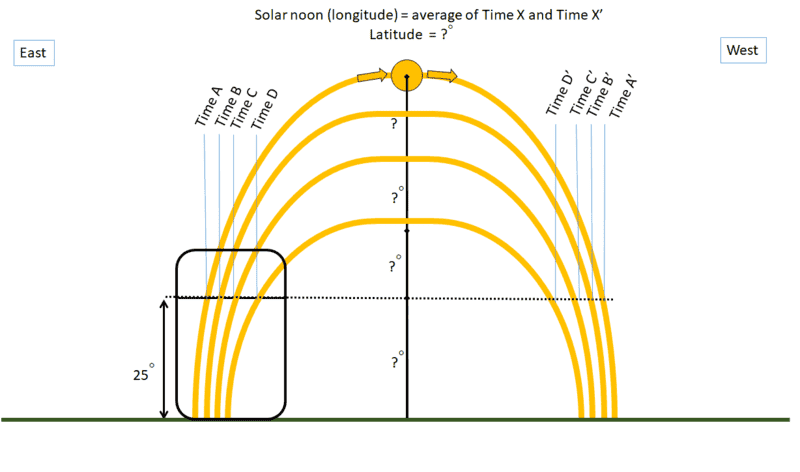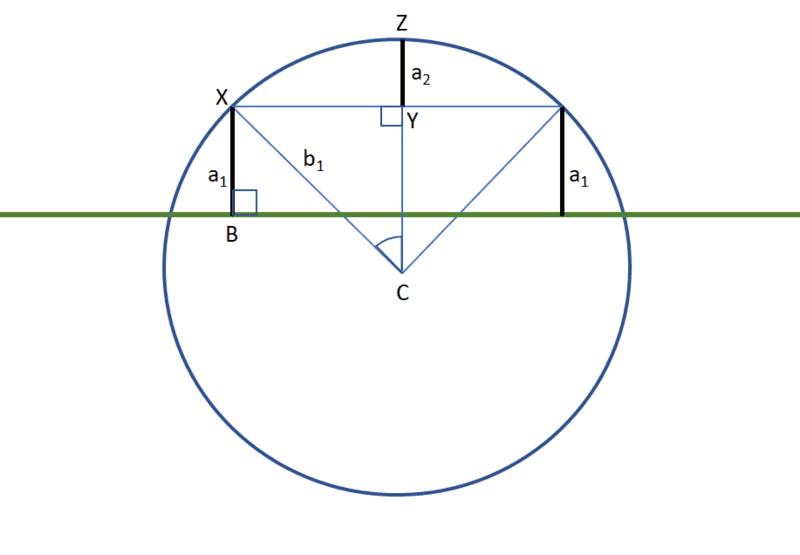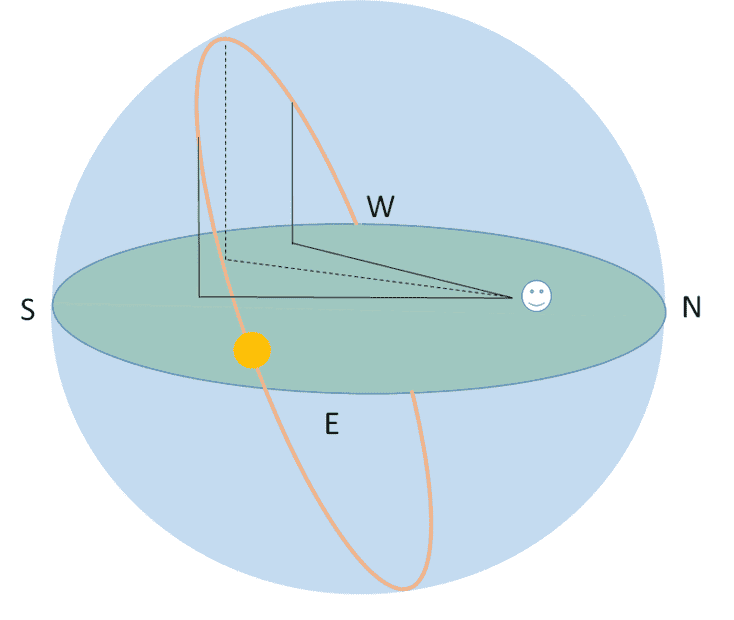# Can we predict the path of the Sun from a single observation point along the transit?

• I
roscoe67
TL;DR Summary
celestial navigation question: can one predict the path of the sun over a day by single observation along the transit?
Hi. I have this idea that might be useful for celestial navigation. If you were on a random place on the Earth, and you had a way of measuring the altitude of the sun above the horizon, at a precise moment, could you then predict the complete path, such that you could determine the altitude at solar noon (the highest position of the sun in that day), and thus calculate latitude (adjusting for solar declination)?

In other words, let's say you set your sextant to 25 degrees above the horizon. If you knew when the sun crossed that altitude (let's say to the second), could you then calculate what would be the sun's highest point?

One thing you would have in this equation would be longitude - you could use the fixed sextant to find the time that the sun passes 25 degrees on it way up and down - averaging the time crossing 25 degrees ascending and descending would give you solar noon, and hence (adjusting for equation of time), longitude.

Let's also assume you know the date.

Is this possible to do?

Thanks!

the idea:WernerQH
If you knew when the sun crossed that altitude (let's say to the second), could you then calculate what would be the sun's highest point?
No, not if you are on a "random place on the Earth". A single measurement of altitude defines only a circle on which the ship's position must lie. But with two measurements you can determine your position. Captain Thomas Sumner discovered this in 1837, you can look up "Sumner line" in Wikipedia.

•russ_watters
roscoe67
No, not if you are on a "random place on the Earth". A single measurement of altitude defines only a circle on which the ship's position must lie. But with two measurements you can determine your position. Captain Thomas Sumner discovered this in 1837, you can look up "Sumner line" in Wikipedia.
Thanks for the response. We can know a little more in this instance. With the second sight (at the same altitude, as the sun descends), we know some parameters of the sun's path. The sun follows an ellipse, and with two sights, can we not infer the shape of the sun's path and, in some way, predict the noon altitude?

This would allow the use of a Briss-style (non-adjustable) sextant for noon sights.

WernerQH
The sun follows an ellipse, and with two sights, can we not infer the shape of the sun's path and, in some way, predict the noon altitude?
It's not an ellipse, but a circle on the celestial sphere. With some spherical trigonometry you can of course calculate the latitude ## \phi ## from the two observations, and deduce the noon altitude ## 90° - \phi + \delta ##.

Determining position as the intersection of two Sumner lines is more flexible. You don't need to wait for the sun to reach the exact same altitude again. (If you are unlucky, it might disappear behind some clouds just at that moment!)

•russ_watters
You still can't measure your longitude unless you know the time relative to some fixed location. If you only know your local time, you don't have enough information. Determining longitude at sea was a major problem, which was solved by having ship's chronometers that were accurate enough so that you knew the time in London.

•Klystron and russ_watters
roscoe67
It's not an ellipse, but a circle on the celestial sphere. With some spherical trigonometry you can of course calculate the latitude ## \phi ## from the two observations, and deduce the noon altitude ## 90° - \phi + \delta ##.

Determining position as the intersection of two Sumner lines is more flexible. You don't need to wait for the sun to reach the exact same altitude again. (If you are unlucky, it might disappear behind some clouds just at that moment!)

OK, see if this makes sense. In the image below, the green line is the horizon. The blue circle is the Sun's path above and below the horizon.

The altitude ascending and descending is a1 (also line BX). If we know the radius of the circle that the Sun follows, then we can calculate the angle XCY as half the time the sun took to cross between the two observed altitudes. Knowing the hypotenuse is the radius, that allows us to calculate the leg CY with good old SOHCAHTOA. That means we can subtract CY from the radius and get YZ. Add YZ to leg BX and you have the altitude of the sun.

There is probably a more elegant way, but that is what I doodled out. However, part of what would make this hard is knowing the radius in a unit that is comparable to the observed altitudes. Fiddling with Stellarium, I can see that the observed radius changes by day.#### Attachments

roscoe67
You still can't measure your longitude unless you know the time relative to some fixed location. If you only know your local time, you don't have enough information. Determining longitude at sea was a major problem, which was solved by having ship's chronometers that were accurate enough so that you knew the time in London.
Yes, in this thought experiment you have a chronometer, not just a stopwatch. I would synch mine to Solar Noon at Greenwich to make longitude calculation easier.

Homework Helper
Gold Member
I'm not sure if you could figure out if you were in the Northern or Southern hemisphere though, with one and only one measurement, plus the chronometer measurement. I mean, sure, you'd be able to figure it out easily enough just by waiting a short time to see which way the Sun is moving (from left to right or from right to left). But that is technically another measurement.

roscoe67
I'm not sure if you could figure out if you were in the Northern or Southern hemisphere though, with one and only one measurement, plus the chronometer measurement. I mean, sure, you'd be able to figure it out easily enough just by waiting a short time to see which way the Sun is moving (from left to right or from right to left). But that is technically another measurement.
Yes, hopefully our theoretical navigator can suss that out. But true, it would have to be made explicit. Close to the Equator it would be tricky I would guess.

Last edited:
Hornbein
I'm not sure if you could figure out if you were in the Northern or Southern hemisphere though, with one and only one measurement, plus the chronometer measurement. I mean, sure, you'd be able to figure it out easily enough just by waiting a short time to see which way the Sun is moving (from left to right or from right to left). But that is technically another measurement.
The Sun rises in the east and sets in the west in all non-polar latitudes.

roscoe67
I worked it out on paper from Stellarium and it is a bit off - about 4 degrees. I am guessing the radius (in degrees) of the Sun's orbit and the visible arc angles are just not aligning. the problem, as I see it, is that the Sun moves in an apparent ellipse, so circular trig won't do it.

Last edited:
WernerQH
There is probably a more elegant way, but that is what I doodled out. However, part of what would make this hard is knowing the radius in a unit that is comparable to the observed altitudes.
You should really learn spherical trigonometry to get this straight.
I am guessing the radius (in degrees) of the Sun's orbit and the visible arc angles are just not aligning. the problem, as I see it, is that the Sun moves in an apparent ellipse, so circular trig won't do it.
There is no perfect way of mapping a sphere on to a plane. There is one method, called stereographic projection, that maps circles on the sphere to circles in the plane. But it does not preserve distances, so you can't directly read off the quantities of interest.

Perhaps the best way for you to proceed, is to take the result from spherical trigonometry $$\sin h = \sin \phi \sin \delta + \cos \phi \cos \delta \cos \tau$$ for the altitude ## h ## and solve for the latitude ## \phi ##. For the hour angle ## \tau ## you need of course both observations: half of the time interval between the first and the second. (## \delta ## is the declination of the sun, which we assume known.)

roscoe67
You should really learn spherical trigonometry to get this straight.

There is no perfect way of mapping a sphere on to a plane. There is one method, called stereographic projection, that maps circles on the sphere to circles in the plane. But it does not preserve distances, so you can't directly read off the quantities of interest.

Perhaps the best way for you to proceed, is to take the result from spherical trigonometry $$\sin h = \sin \phi \sin \delta + \cos \phi \cos \delta \cos \tau$$ for the altitude ## h ## and solve for the latitude ## \phi ##. For the hour angle ## \tau ## you need of course both observations: half of the time interval between the first and the second. (## \delta ## is the declination of the sun, which we assume known.)

Any excuse to dive further into trig is fine with me - it is one of my favorite forms of math. It will take me a while to parse out your formula. Unfortunately, I tend to work visually for trig problems. I just thought an ellipse because it seems like it might be easier to solve. We see the sun's path as elliptical, no? Or, rather half an ellipse - a parabola?Last edited: